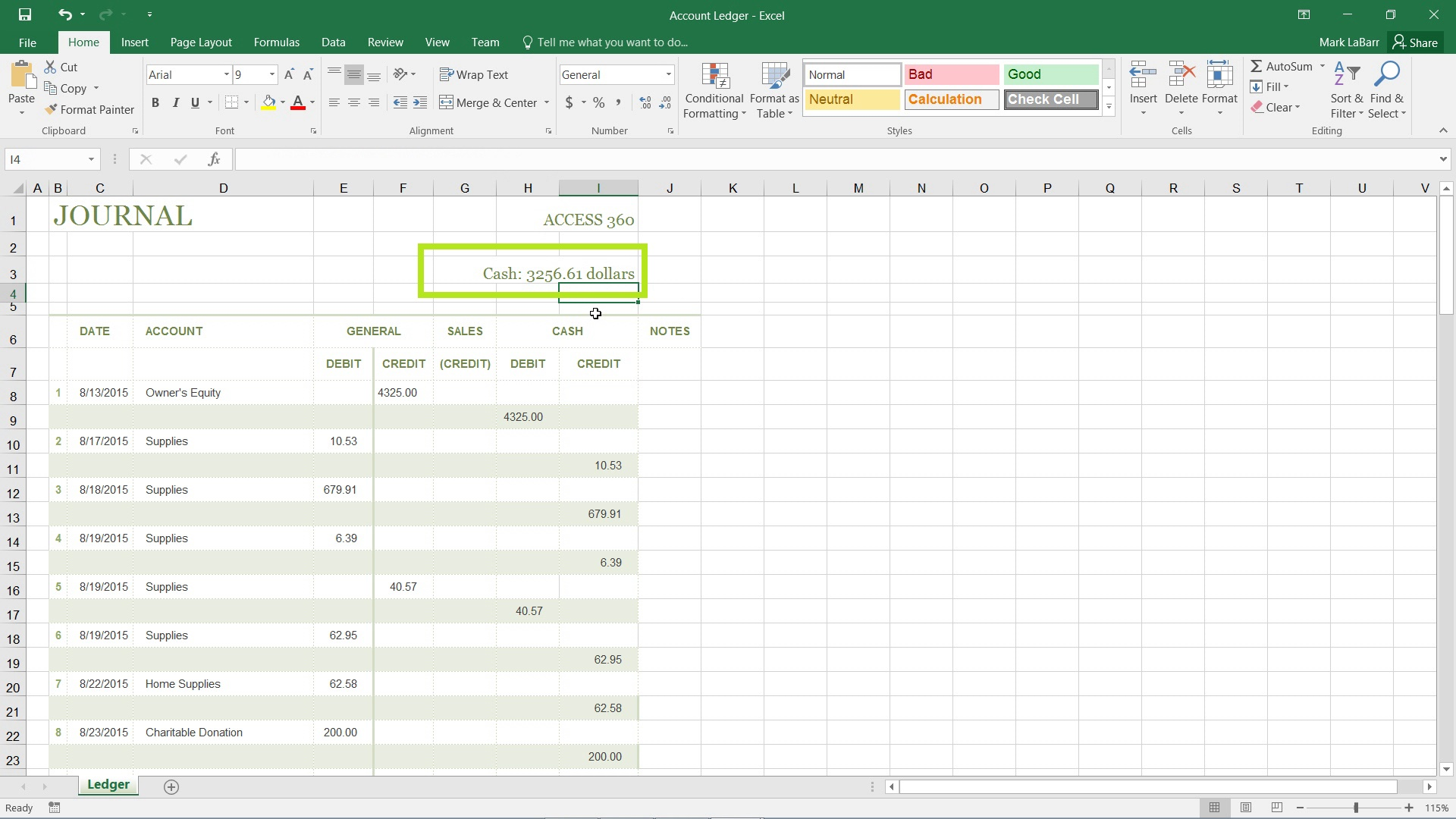## How to Add Text into an Excel 2016 Formula

In this tutorial, we’re going to show you how to add text to an Excel 2016 formula.

First, start off like any other formula, with an equals sign. Text is a string value, thus it must be enclosed in quotation marks. The example shown below uses the formula: [=”Cash: “].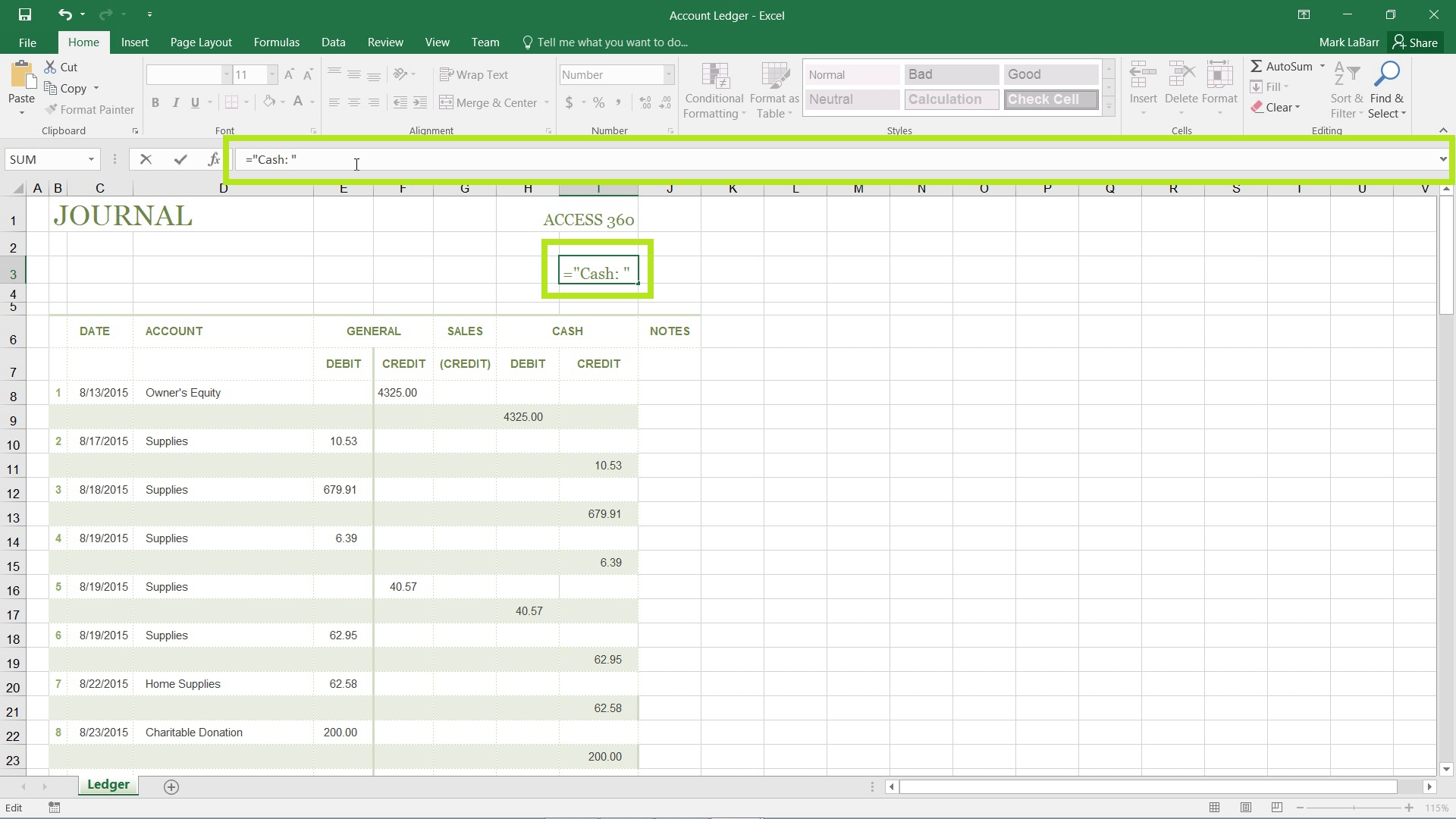To add an actual calculation to the formula after the string value, you must use the ampersand symbol (&) to concatenate, or add, the calculation on at the end of the formula.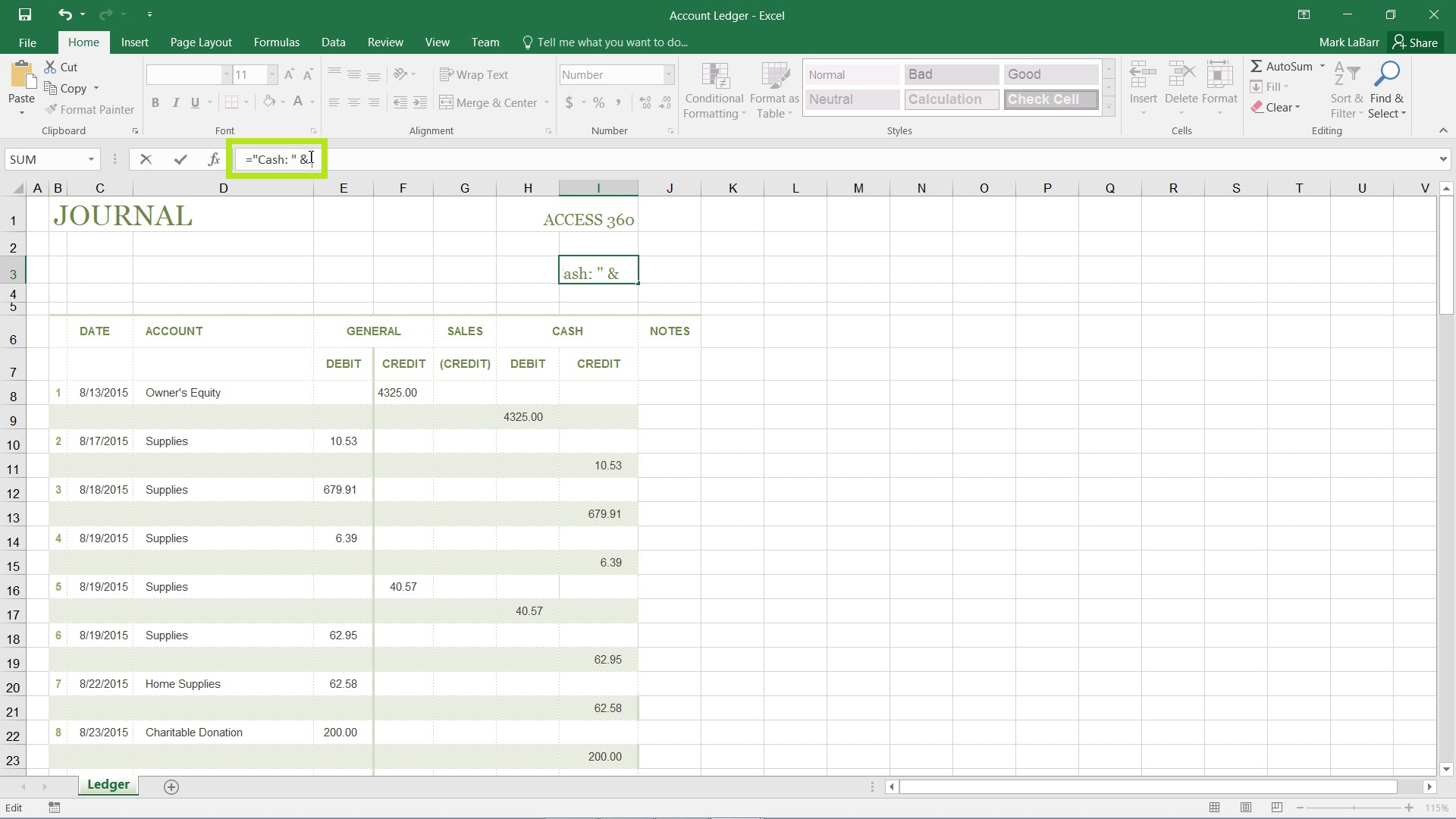The below example adds in the actual calculation.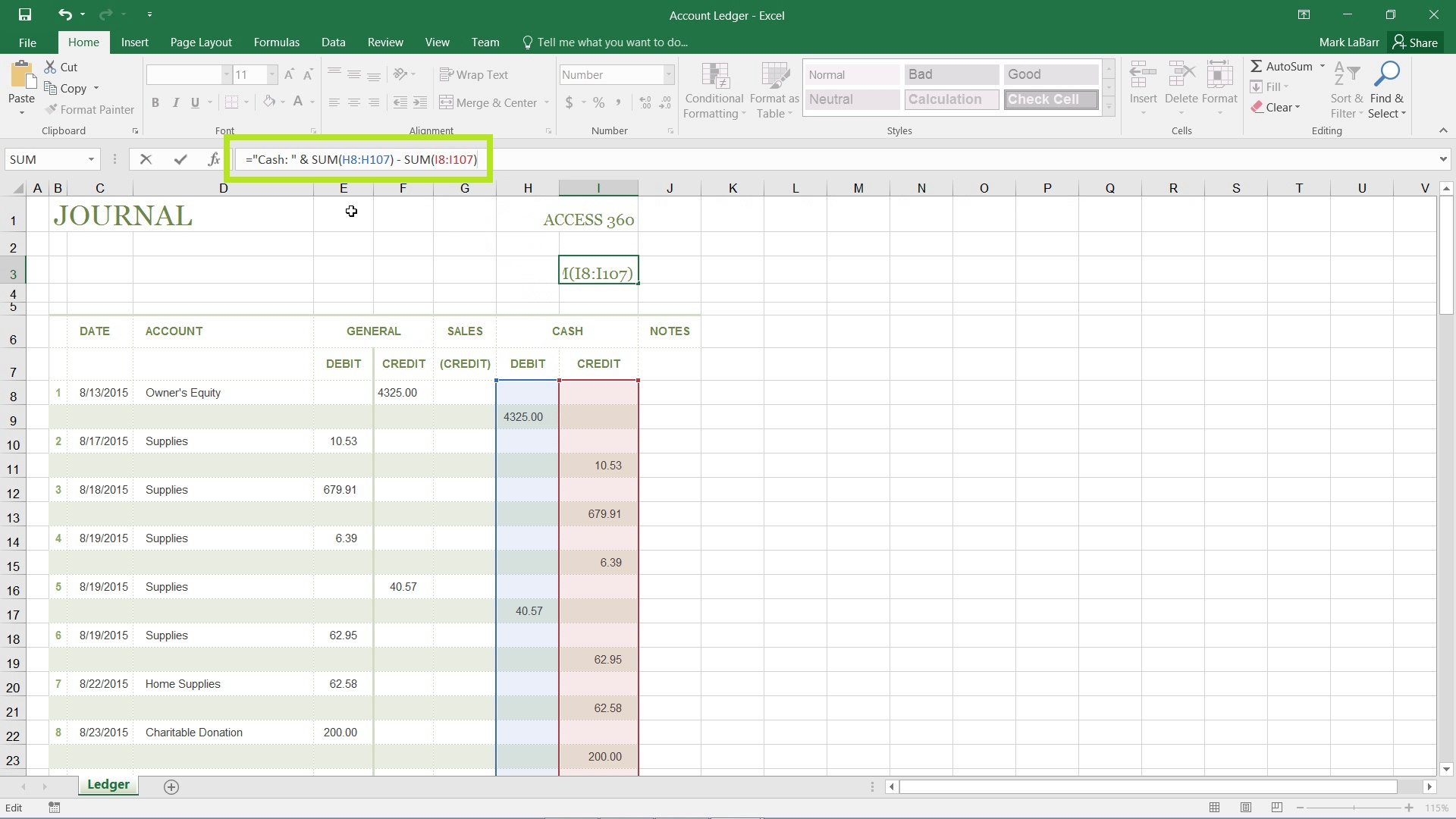To add an extra element to the formula, more text for example, simply throw in another ampersand (&) symbol before the new text. The full formula shown below is as follows: [=”Cash: “ & SUM(H8:H107) – SUM(I8:I107) & “ dollars”]. Make sure to include the spaces in the string values.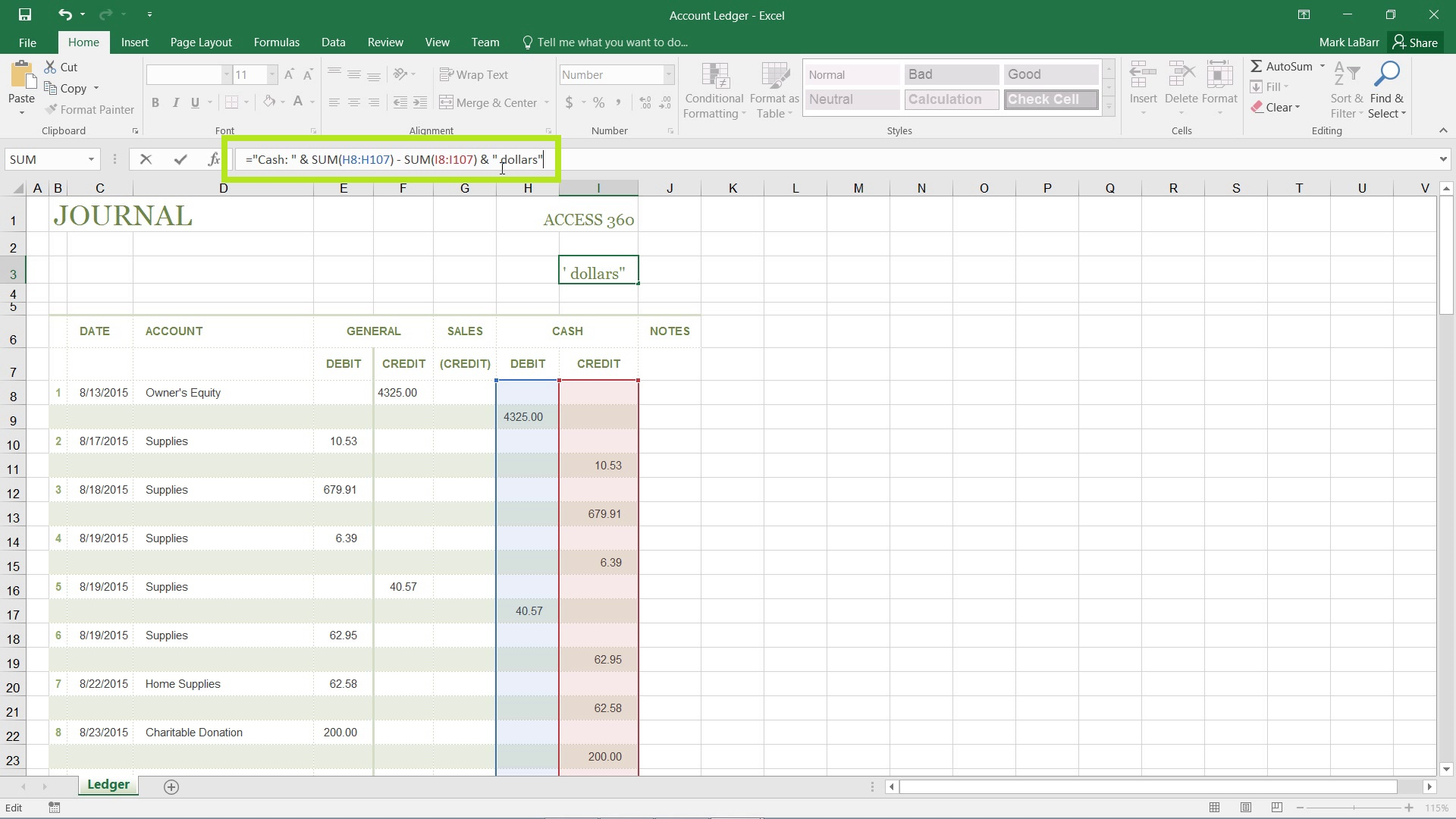The result that shows after hitting Enter, or selecting a different cell should be as shown below. A calculation in between two string values that have been concatenated on.